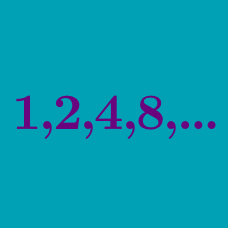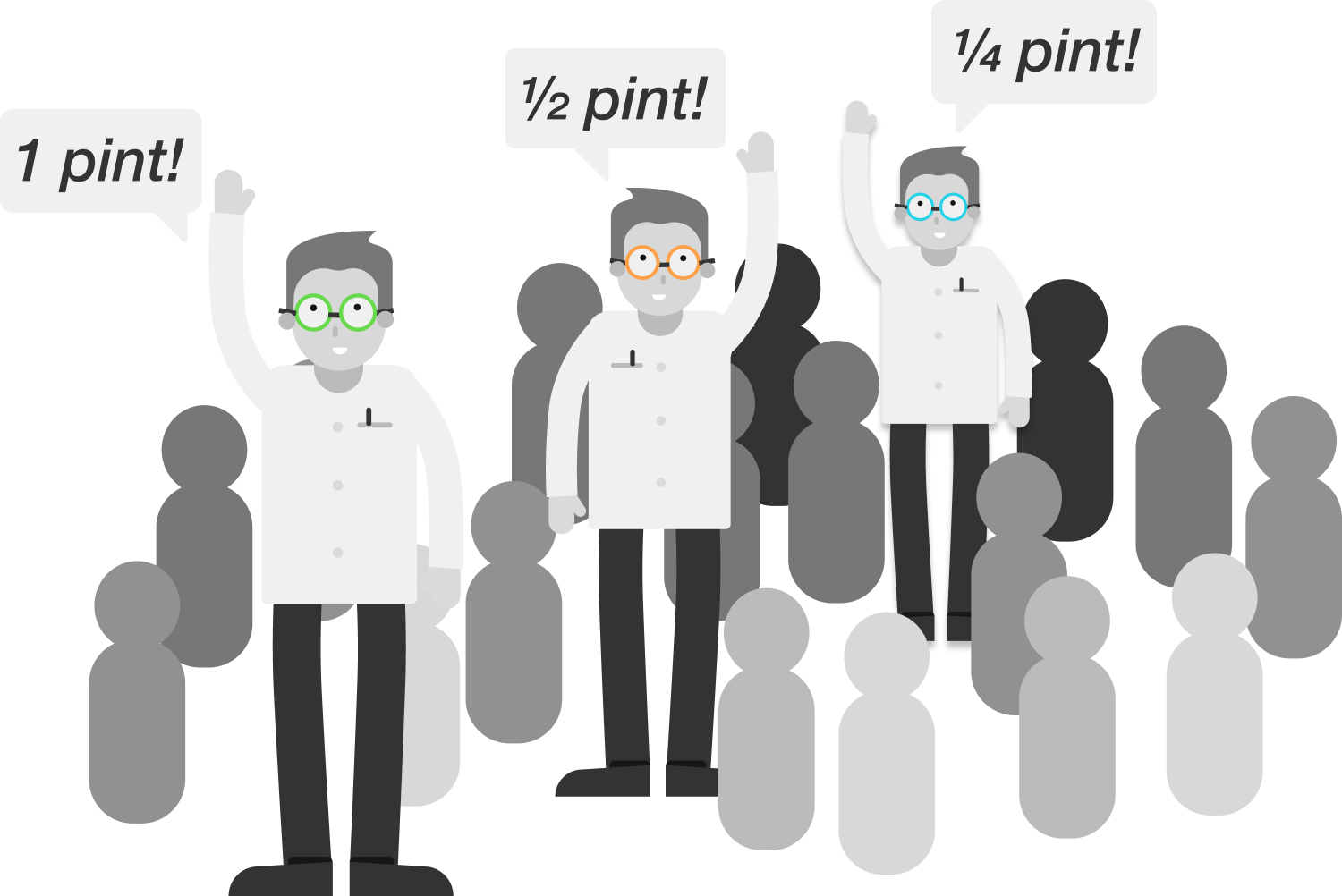Algebra

# Geometric Progressions: Level 2 ChallengesAn infinite crowd of mathematicians enters a bar. The first one orders a pint, the second one orders a half pint, the third one orders a quarter pint, and so on.

The bartender interrupts, "I get it!" – then pours the order for all of the mathematicians. How many pints does the bartender pour?

$\large 1+x + x^2 + x^3 + x^4 + \ldots = 15 \qquad, \qquad x = \ ?$

$1+2 \cdot 2+ 3 \cdot 2^2 + 4 \cdot 2^3 + \cdots+ 100 \cdot 2^{99}= \, ?$

$\frac{a}{b}+\frac{a}{b^{2}}+\frac{a}{b^{3}}+\ldots = 4$

$\frac{a}{a+b}+\frac{a}{(a+b)^{2}}+\frac{a}{(a+b)^{3}}+\ldots = \ ?$

If the first three terms of a geometric progression are given to be $$\sqrt2+1,1,\sqrt2-1,$$ find the sum to infinity of all of its terms.

If the answer is in the form of $$\frac{a+b\sqrt c}d$$ for positive integers $$a,b,c,$$ and $$d$$ with $$c$$ square-free, find the minimum value of $$a+b+c+d$$.

×

Problem Loading...

Note Loading...

Set Loading...